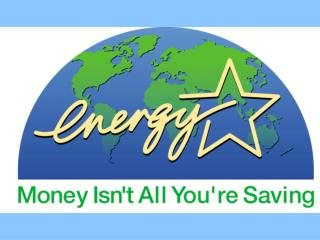# ENERGY - PowerPoint PPT PresentationDownload PresentationENERGY

ENERGYDownload Presentation## ENERGY

- - - - - - - - - - - - - - - - - - - - - - - - - - - E N D - - - - - - - - - - - - - - - - - - - - - - - - - - -
##### Presentation Transcript

1. ENERGY

2. Class Starter 1) What is energy? 2) Is energy a form of matter? Explain your answer. 3) Try to name 10 different forms of energy.

3. 1) What is energy? • Energy is the ability to do work. • Examples of work accomplished by energy: • Making objects move • Making new chemical compounds • Heating substances. .

4. 2) Is energy a form of matter? Explain your answer. Energy is not considered to be matter. Matter is anything that has a mass and takes up space (volume). Energy does not have a measureable mass or volume.

5. 1) Potential Energy • The energy an object possesses because of its position above a surface. • Potential energy is converted to kinetic energy (moving energy) when an object is dropped. Examples: • a pencil on a table • a roller coaster waiting to drop

6. Potential energy being converted to kinetic energy

7. 2) Kinetic Energy The energy of objects in motion. Examples: • A bicycle in motion • Amoving car

8. 2) Kinetic Energy

9. 3) Elastic Energy The energy an object possesses when its shape is changed through compression or compression. Examples: A stretched elastic band is stretched or a compressed spring both store elastic energy.

10. 3) Elastic Energy

11. 4) Radiant Energy Energy that allows us to see objects around us Examples: The sun, lights

13. 5) Electrical Energy Energy carried by electrons traveling through a wire Examples: Electrical energy is used to power most household appliances

14. 5) Electrical Energy

15. 6) Magnetic Energy Energy stored in the force of 2 magnets’ attraction towards one another Example: Magnets

16. 6) Magnetic Energy

17. 7) Thermal (heat) Energy The internal energy of an object due to the motion of its particles (atoms and molecules) of matter. The faster the particles move, the warmer the matter can get. Example: Hot water has a lot of thermal energy because it’s particles move really fast.

18. 7) Thermal (heat) Energy

19. 8) Chemical Energy Energy that is released during a chemical reaction Examples: • The energy in gas turns car engines. • Your body uses chemical energy contained in food.

20. 8) Chemical Energy

21. 9) Nuclear Energy Energy contained by the nucleus of atoms Example: Uranium contains nuclear energy which is used to create energy in nuclear power pants.

22. 9) Nuclear Energy

23. 10) Sound Energy Energy produced when mater vibrates Example: Sound waves emitted by a speaker vibrate air particles which make the small bones of your inner ear move. This sends a message to your brain which allows you to hear.

24. 10) Sound Energy

25. Summary of Different Forms of Energy